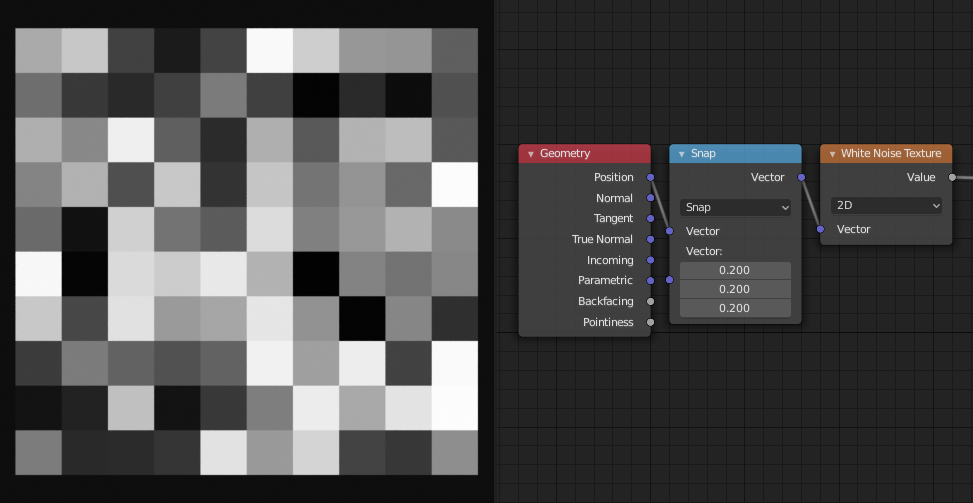# White Noise Texture Node

Note

This node is ported from shader nodes. The manual and images are referencing the shader version of the node. This node accepts field inputs and outputs. When not connected the Vector input has an implicit `position` attribute value.

The White Noise Texture node returns a random number based on an input Seed. The seed can be a number, a 2D vector, a 3D vector, or a 4D vector; depending on the Dimensions property. The output number ranges between zero and one.

## Inputs

The inputs are dynamic, they become available if needed depending on the node properties.

Vector

Vector used as seed in 2D, 3D, and 4D dimensions.

W

Value used as seed in 1D and 4D dimensions.

## Properties

Dimensions

The dimensions of the space to evaluate the noise in.

1D

The W input is used as seed.

2D

The X and Y components of the Vector input are used as seed.

3D

The Vector input is used as seed.

4D

Both the Vector input and the W input are used as seed.

## Outputs

Value

Output random value.

Color

Output random color.

## ExamplesGenerating cell noise using the Snap vector operation and the White Noise node.